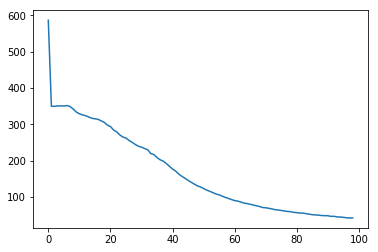# Validation loss increasing instead of decreasing

Hi Guys,
I am very new at pytorch and deep learning. I am trying to use a CNN+LSTM structure for a regression problem. Where input is time series data (1,5120). CNN is for feature extraction purpose. My output is (1,2) vector. Even though my training loss is decreasing, the validation loss does the opposite. I tried several things, couldn’t figure out what is wrong.
Can you give me any suggestion?
here is my network. the MSE loss plots

class ConvNet(nn.Module):
def init(self):
super(ConvNet, self).init()
self.layer1 = torch.nn.Conv1d(in_channels=1, out_channels=5, kernel_size=3, stride=2,bias=0)
self.pool = nn.MaxPool1d(3, stride = 2)
self.layer2 = torch.nn.Conv1d(in_channels=5, out_channels=10, kernel_size=3, stride=2,bias=0)
self.drop_out = nn.Dropout2d()
self.fc1 = nn.Linear(512, 512,bias=True)

``````def forward(self, x):

out = self.layer1(x)
out = F.relu(out)
out = self.pool(out)
out = self.layer2(out)
out = F.relu(out)
out = out.reshape(out.size(0), -1)
'''
out = self.drop_out(out)

out = self.fc1(x)
out = F.relu(out)
'''
return out
``````

class Combine(nn.Module):
def init(self):
super(Combine, self).init()
self.cnn = ConvNet()
self.lstm1 = nn.LSTM(
input_size=639,
hidden_size=128,
num_layers=1,
bidirectional = True,
batch_first=True)
self.drop_out = nn.Dropout()
self.lstm2 = nn.LSTM(
input_size=256,
hidden_size=100,
num_layers=1,
bidirectional = False,
batch_first=True)
self.batchnorm = nn.BatchNorm1d(10 *100,affine = False)
self.fc = nn.Linear(1000,2, bias=True)
def forward(self, x):
xin = x.view(x.size(0),1,-1)
cn_out = self.cnn(xin)

``````    c_in = cn_out.view(batch.size(0), 10, 639)

h0 = Variable(torch.zeros(2, batch.size(0), 128)).cuda()
c0 = Variable(torch.zeros(2, batch.size(0), 128)).cuda()

outputs, (ht, ct) = self.lstm1(c_in,(h0,c0))
outputs = self.drop_out(outputs)

h0 = Variable(torch.zeros(1, batch.size(0), 100)).cuda()
c0 = Variable(torch.zeros(1, batch.size(0), 100)).cuda()

outputs = outputs.view(batch.size(0), 10,256)
out3,(ht, ct)  = self.lstm2(outputs)
out = self.fc(out3.contiguous().view(batch.size(0), -1))
return out
``````